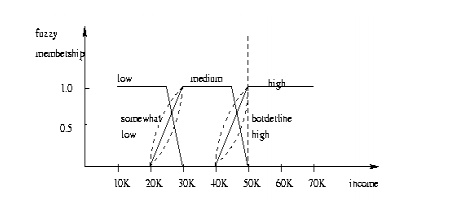Home | | Data Ware Housing and Data Mining | Other Classification Methods

# Other Classification Methods

Genetic Algorithm: based on an analogy to biological evolution

Other Classification Methods

Genetic Algorithms

o     Genetic Algorithm: based on an analogy to biological evolution

o     An initial population is created consisting of randomly generated rules

·         Each rule is represented by a string of bits

·        E.g., if A1 and ¬A2 then C2 can be encoded as 100 o If an attribute has k > 2 values, k bits can be used

o     Based on the notion of survival of the fittest, a new population is formed to consist of the fittest rules and their offsprings

o     The fitness of a rule is represented by its classification accuracy on a set of training examples

o     Offsprings are generated by crossover and mutation

o     The process continues until a population P evolves when each rule in P satisfies a prespecified threshold

o     Slow but easily parallelizable

Rough Set Approach:

o     Rough sets are used to approximately or ―roughly‖ define equivalent classes

o     A rough set for a given class C is approximated by two sets: a lower approximation (certain to be in C) and an upper approximation (cannot be described as not belonging to C)

o   Finding the minimal subsets (reducts) of attributes for feature reduction is NP-hard but a discernibility matrix (which stores the differences between attribute values for each pair of data tuples) is used to reduce the computation intensityFigure: A rough set approximation of the set of tuples of the class C suing lower and upper approximation sets of C. The rectangular regions represent equivalence classes

Fuzzy Set approaches

o     Fuzzy logic uses truth values between 0.0 and 1.0 to represent the degree of membership (such as using fuzzy membership graph)

o     Attribute values are converted to fuzzy values

e.g., income is mapped into the discrete categories {low, medium, high} with fuzzy values calculated

o     For a given new sample, more than one fuzzy value may apply

o     Each applicable rule contributes a vote for membership in the categories

o   Typically, the truth values for each predicted category are summed, and these sums are combinedStudy Material, Lecturing Notes, Assignment, Reference, Wiki description explanation, brief detail
Data Warehousing and Data Mining : Association Rule Mining and Classification : Other Classification Methods |